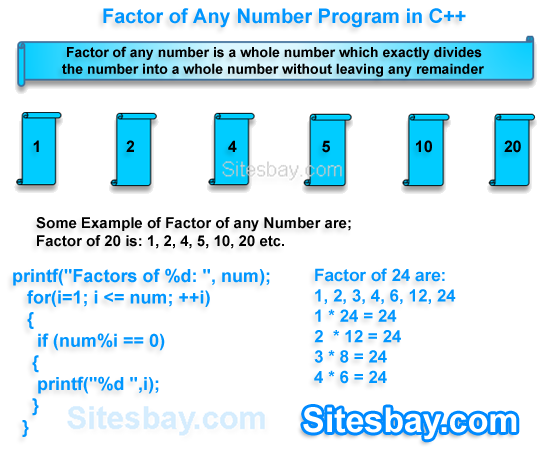# Factor of Number Program in C

## Find Factor of any Number Programs in C

Factor of any number is a whole number which exactly divides the number into a whole number without leaving any remainder. For example: 3 is a factor of 6 because 3 divides 6 exactly leaving no remainder and is factor of every number beacuse every number is divisible by 1.

Here we find factor of any given number by user, Let's start write a C program to input a number from user and find all factors of the given number using for loop. See genera example of factor of 15 is: 1, 3, 5, 15 and factor of 20 is: 1, 2, 4, 5, 10, 20### Logic to find all factors of a number in C

• Receive any number from user and store in any veriable num
• Execute a loop from 1 to num, increment 1 in each iteration. The loop structure should look like for(i=1; <=num; i++)
• For each iteration inside loop check current counter loop variable i is a factor of num or not. Check num is divisible by i and reminder is zero.
• Check number
• If i is a factor of num then print the value of i.

## Factor program in C Using For Loop

```#include<stdio.h>
#include<conio.h>

void main()
{
int num, i;
clrscr();
printf("Enter a positive integer: ");
scanf("%d",&num);

printf("Factors of %d are: ", num);
for(i=1; i <= num; ++i)
{
if (num%i == 0)
{
printf("%d ",i);
}
}
getch();
}
```

## Output

```Enter a positive integer: 15
Factors of 15 are: 1 3 5 10
```

## Output 2

```Enter a positive integer: 30
Factors of 30 are: 1 2 3 4 5 6 10 12 15 30
```

## Output 3

```Enter a positive integer: 60
Factors of 60 are: 1 2 3 4 5 6 10 12 15 20 30 60
```

## Factor program in C Using While Loop

```#include<stdio.h>
#include<conio.h>

void main()
{
int number, i=1;
clrscr();
printf("Please Enter number to Find Factors: ");
scanf("%d",&number);

printf("Factors of %d are: ", number);

while (i <= number)
{
if(number%i == 0)
{
printf("%d  ",i);
}
i++;
}

getch();
}
```

## Output

```Please Enter number to Find Factors: 15
Factors of 15 are: 1 3 5 10
```

WIFI CCTV Camera